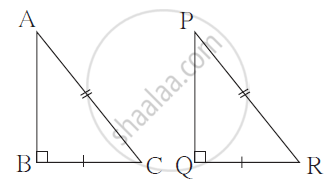# In the Figure, Parts of the Two Triangles Bearing Identical Marks Arecongruent. State the Test by Which the Triangles Are Congruent. - Geometry

In the figure, parts of the two triangles bearing identical marks are
congruent. State the test by which the triangles are congruent.#### Solution

Δ ABC and ΔPQR are congruent by hypotenuse side test.

Concept: Similarity of Triangles
Is there an error in this question or solution?